## Deriving Formal Parameters for Comparing Knowledge Elicitation Techniques Based on Mathematical Functions.

Rodrigo Martinez-Bejar*, Richard Benjamins**, Fernando Martin*** and Victor Catillo*

*Spanish Council for Scientific Research - CEBAS, Avda. La Fama, 1, C.P. 30080, Murcia, Spain. Email: rodrigo,victor@natura.cebas.csic.es
**Department of Social Science Informatics, University of Amsterdam, Roetersstraat 15, 1018 WB Amsterdam, The Netherlands. Email: richard@swi.psy.uva.nl
***Department of Computing Sciences, University of Murcia, Murcia, Spain. Email:fmartin@dif.um.es

The knowledge elicitation process has a considerable influence on the quality of the knowledge-based system to be developed. Moreover, because knowledge elicitation is an important cost-determining factor, a good elicitation technique should reveal the relevant knowledge in the minimum amount of time possible. In this paper, we derive a set of formal parameters, based on mathematical functions, for comparing knowledge elicitation techniques. We use the landscape study task (a sub-task of environmental planning) as an illustrating example throughout.

### 1 Introduction

Although, in the past decade, several methodologies for Knowledge Acquisition (KA) have been developed, the knowledge elicitation process is still more an art than a science. There are many different elicitation techniques (Cooke, 1994) and selecting the "right" technique in a particular situation is not trivial. Moreover, knowledge elicitation involves skilled people and this makes it an important cost-determining factor in the development process of a knowledge-based system (KBS). There are two important factors to consider here: (1) the kind of knowledge the technique elicits and (2) the time needed to apply the technique. In a particular knowledge engineering situation, one would, ideally, use an elicitation technique that provides all the relevant knowledge in the minimum amount of time possible.

Previous work has shown that there exists a relationship between, on the one hand, the elicitation technique used to gather data and, on the other hand, the resulting model of expert knowledge (Nisbett and Wilson, 1977; Berry, 1987; Gammack, 1992). This implies that we should not economise by opting for a cheaper technique if this technique cannot elicit the relevant knowledge. However, we can optimise the elicitation process by trying to minimise the time spent on the elicitation process. Previous work on knowledge elicitation techniques has focused on empirical comparisons (Burton, Shadbolt, Rugg, and Hedgecock, 1990).

The aim of this paper is to formally characterise elicitation techniques concerning the required time of their application in relation to a quantitative measure of the knowledge they provide. The aim is achieved by deriving a set of formal parameters for comparing elicitation techniques, based on a normalisation of the underlying knowledge. The ideas in the paper are developed, based on, and will be illustrated with an existing KBS project for environmental planning in Spain.

In the overall development process of a KBS, this work has to be situated in the first stage, namely in the knowledge elicitation phase. Modern knowledge acquisition methodologies such as KADS (Wielinga, Schreiber, and Breuker, 1992), VITAL (O'Hara, Motta, and Shadbolt, 1994) and PROTÉGÉ (Puerta, Egar, Tu, and Musen, 1992) are concerned with conceptual modelling (based on the elicited knowledge) and with later stages in the KBS development process. Therefore, the result of our work can be of use for each of these methodologies.

The structure of the paper is as follows. Section 2 offers a brief overview of the main factors which influence the duration of knowledge elicitation processes. Also, assumptions to be taken into account in the rest of the paper in relation to these factors are put forward. In Section 3, we formally describe characteristics of elicited knowledge by means of mathematical funstions, and we introduce an algorithm for sampling the amount of knowledge. In Section 4, we present a design of parameters for comparing knowledge elicitation techniques with respect to their respective knowledge samples obtained after applying the mentioned algorithm. Section 5 shows an example where the theory introduced in the previous sections is used to compare the structured interview method with the unstructured one for an assessment problem. Finally, in Section 6 we present conclusions.

### 2 The Elicitation-Process Handicap

One of the reasons that it is expensive to develop KBSs, is that the development process involves considerable time and effort of knowledge engineers and domain experts, and such people are costly. In this sense, the knowledge elicitation process (KEP) is an important cost-determining factor in the whole knowledge acquisition process. This is one of the reasons that some researchers try to do the job without knowledge engineers. For example, Ripple Down Rules (Kang, Compton, and Preston, 1995) allow to perform knowledge acquisition without knowledge engineers; human experts directly input the rules which are relevant to solve the problem (although at the expense of the structure of the rule base). Other research groups focus on automatic generation of elicitation tools in which the domain experts can directly put their knowledge. Examples include SALT and MOLE (Marcus, 1988), PROTÉGÉ (Puerta, Egar, Tu, and Musen, 1992), and DIDS (Runkel and Birmingham, 1993).

However, in many situations, interaction between a knowledge engineer and domain expert remains indispensable. In order to keep the costs as low as possible, we want to minimise the time of the KEP, while keeping its content. We hope to achieve this goal by selecting the adequate elicitation technique in different situations. To do that, first, we have to identify the possible causes of the duration of the KEP. Hart (1986) and Cooke (1994), identify a number of them, which we briefly review in the following paragraphs.

The number of experts Some problems require several experts in different areas. For example, the environmental planning task requires knowledge of geology, zoology, botanical, ecology, economy, hydrology, and forestry engineering. It is not likely to find all these skills in one person, but in several experts. This implies that each of the experts will have a particular, probably distinct, language for describing his or her domain.

As a consequence of this variety of languages, redundancies and inconsistencies can be introduced and, hence, it will increase the duration of the KEP. For example, consider that there are two experts who participate in the environmental planning project whose objective is to evaluate the visual fragility of a landscape belonging to a particular forest. Let us assume that one of the experts is a zoologist (Z) and the other a botanist (B). Suppose that expert Z states that "if the diet of the rabbits of the forest is constituted by singular plants then the visual fragility of the forest is high", while expert B asserts that "if there are rare plants, the fragility of the forest could be high". After having analysed the respective antecedents of the above assertions, it may arise that the verbs to constitute and to exist are synonyms, as well as the concepts rare plants and singular plants.

The domain In some domains, the elicited knowledge may become invalid before it has been made operative in a KBS. This occurs for example when the basis of the theory supporting the principles of expert actions changes during the development process. In the environmental planning project, this could happen when the corresponding policy of the government changes during the KEP (e.g., because of a change of president).

Other domain related factors that complicate the KEP include: (1) the needed knowledge is strictly confidential (e.g., for strategic reasons); (2) the knowledge required to solve the problem is expensive to obtain (e.g., because experts are not readily available due to long travel distances); (3) the knowledge may be complex (e.g., because the experts reason with uncertainty and with linguistic hedges (Hwang, 1995)) such as very, high, more, etc.

The knowledge engineer The knowledge engineer, as a person with certain characteristics, is also an important factor for the time needed for the KEP. Welbank (1983) identifies a number of interpersonal skills which a knowledge engineer ideally should possess, including self-confidence, tact and diplomacy, and intelligence; versatility and inventiveness; empathy, patience and persistence. Moreover, a knowledge engineer has to know about domain and programming knowledge, and about elicitation techniques, machine learning techniques, expertise modelling, knowledge representation methodologies and available commercial shells.

The context The context relates to the equipment (e.g. tape recorder, video) used for interviewing the experts, the environmental characteristics of the location where the KEP occurs, etc. For example, if a knowledge engineer does not have a tape recorder or a video recorder at his or her disposal, and interviews have to be taken, it will take more time to record experts' answers in general.

2.1 Assumptions

With respect to the KEP, and taking into account the just mentioned factors, in the rest of the paper we make the following assumptions in order to keep our problem manageable.

Assumption 1 The kind of problems to be solved concern domains in which knowledge of different areas is needed. We assume that these domains may be structured in such a way that each area corresponds to a separate task as distinguished in the KADS methodology (Wielinga, et al., 1992). Thus, we assume an interdisciplinary problem, where each individual discipline involves a separate task.

Assumption 2 There are Ne available experts, Ne > 1, in such a way that they cover all the disciplines underlying the tasks whose expert knowledge needs to be elicited. Moreover, there is at least one expert per involved discipline. In addition, we will assume Ne is constant during the KEP. It is clear that using the same human expert for a specific task during the entire KEP facilitates consistency among the segments of knowledge obtained with elicitation techniques. We also assume that experts are available whenever they are needed during the KEP.

Assumption 3. Because we aim at comparing knowledge elicitation techniques, we assume that all known techniques from the literature, as well as the equipment necessary to carry out each of such techniques, are available. Moreover, we assume that the knowledge engineer knows how to apply each elicitation technique.

Assumption 4 Most knowledge elicitation techniques require the active participation of elicitors. Analogue to keeping the same human experts during the KEP, we assume the same elicitor during the KEP.

Assumption 5 Each knowledge elicitation session is carried out in such a way that the addition of knowledge during a knowledge elicitation session does not invalidate knowledge elicited in previous sessions.

A problem that satisfies the assumptions mentioned above is referred to as a knowledge engineering solvable problem (KE-solvable problem).

In this paper, we are interested in a comparison of knowledge elicitation techniques, which is needed to address questions such as the validity of the techniques (Cooke, 1994). Mathematical analysis provides a rigorous "corpus" to perform such comparison in a formal and systematic way. It can be used to model the process of extracting concepts and relations after each elicitation session. In particular, several functions for quantifying concepts, their attributes and their values can be defined by using mathematical analysis. In the following, we will present a formal framework, built on top of the mentioned assumptions, to derive parameters which can be used to compare knowledge elicitation techniques.

### 3 Accumulation and Finiteness of the Elicited Knowledge

We focus on expert knowledge for solving a KE-solvable problem from a quantitative point of view. More precisely, we are interested in the time spent on eliciting knowledge from human experts and in representing this knowledge in countable entities. The definitions in the following subsection aim at defining, in a step-by-step process, the knowledge function which is the base for deriving the formal parameters later in the paper.

3.1 Knowledge functions

Definition 1: ASS Let Kru be the set of possible decision rules which can be elicited from human experts, and let Kc be the set of semantically different concepts underlying Kru. The Association operator, written ASS, is defined as a function which maps Kru to Kc in order to obtain the semantically different concepts underlying a particular decision rule elicited from some expert.

Definition 2: ISA Given a hierarchy of concepts elicited earlier, the Ascendants, operator, written ISA, is defined as a function which maps Kc to itself in order to obtain the set of ascendant concepts of a concept obtained after applying the ASS operator to a particular decision rule elicited from one or more experts.

Definition 3: PRO Let Kp be the set of properties relative to Kc. The Properties operator, written PRO, is defined as a function which maps the Cartesian product Kru x Kc to Kp in order to obtain for each concept the set of properties involved in a particular rule from which that concept has been obtained (i.e., after applying the ASS operator to the rule under question). For example, by assuming that r is a decision rule elicited from one or more experts, and z is a concept belonging to ASS(r), PRO (r, z) provides the set of properties, which have to do with z, implicitly or explicitly referenced in r. In order to solve possible ambiguity problems, each of the so obtained properties can be written as concept.property, where property = PRO (r, z) and concept = z.

Definition 4: VAL Let Kv be the set of possible values associated to the elements of Kc. The Values operator, written VAL, is defined as a function which maps the Cartesian product Kru x Kc x Kp to Kv. For instance, by assuming that r is a decision rule elicited from one or more experts, that the concept zASS(r), and that the property uPRO(r,z), VAL(r,z,u) provides the value corresponding to the concept z for the property u in such a way that it can explicitly be extracted from r. Possible ambiguity problems can be solved by introducing the notation concept.property.value, where value is equal to VAL(r,z,u); property is the property referenced by value, that is, u; concept is the concept to which value is linked, that is, z.

Due to the way ASS, ISA, PRO and VAL are defined above, their arguments can only consist of single elements, and not of sets of elements. However, sometimes it may be necessary that the functions accept a set of elements as arguments. For example, when dealing with sets of rules or concepts as a whole. In the following, we will extend the definitions in such a way that their arguments can manage sets of elements.

Definition 5: MASS Let R be a non-empty set of expert decision rules. The multiple association operator, written MASS, is defined as follows:, where rj stands for the jth rule belonging to R, j = 1,..,Card(R).

In a analogous way, we can extend the function of ISA.

Definition 6: MISA Let R be a non-empty set of expert decision rules, and let C be equal to MASS(R). The multiple ascendants operator, written MISA, is defined as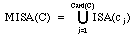, where cj stands for the ith concept belonging to C, j = 1,..,Card(C).

Similarly, we can do the same for PRO. However, for readability reasons, we will do it in a two-step process.

Definition 7: MPRO Let r be an expert decision rule, such that C = ASS(r). The multiple properties operator, written MPRO, is defined as follows: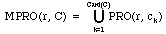, where ck stands for the kth element belonging to C, k = 1,..,Card(C).

Definition 8: EPRO Let R be a non-empty set of expert decision rules, and C be equal to MASS(R). The extended property operator, written EPRO, is defined as follows:, where Cj = ASS (rj), j = 1,..,Card(R).

By proceeding in a similar way as done for the PRO operator, we can extend the definition for VAL.

Definition 9: MPVAL Let r be an expert decision rule, and let c be a concept belonging to ASS(r) such that P = PRO(r,c). The multiple properties value operator, written MPVAL, is defined as follows:, where pi stands for the ith element belonging to P, i = 1, 2, ..., Card(P).

Definition 10: MCVAL Let r be an expert decision rule, and let C be equal to ASS(r) such that P = MPRO(r, C). The multiple concepts value operator, written MCVAL, is defined as follows:, where i stands for the ith element belonging to C, i = 1, 2, ..., Card(C).

Definition 11: EVAL Let R be a non-empty set of expert decision rules, let C be equal to MASS(R) and let P be equal to EPRO(R, C). The extended assessment operator, written EVAL, is defined as follows:, where ri stands for the ith element belonging to R, i = 1, 2, ..., Card(R).

Definition 12: K*(t) Let Kru(t) be the set of decision rules which have been elicited from one or more human experts until the instant t, let Kc(t) be MASS(Kru(t)), and let Kr(t) be equal to the union set MISA(Kc(t))EPRO(Kru(t), Kc(t))EVAL(Kru(t), Kc(t), EPRO(Kru(t), Kc(t))). Using the above terminology, the amount Card(Kc(t)) + Card(Kr(t)), written K*(t), is said to be the cumulative knowledge function until t.

As immediate consequences, the next properties with respect to K*(t) can be established:

1. K*(t) >= 0.

Proof Only two possible situations can arise:

a) t = 0. Before the KEP begins, there is no elicited knowledge and, hence, K*(0) = 0.

b) t > 0. In that case:

K*(t) =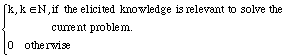2. K*(t) is an increasing, monotonous function.

Proof It is obvious that as more time is consumed by the KEP, the amount of elicited knowledge either increases or remains as a constant, but never decreases.

3.2 Example

Suppose that the following information elicited until ti is available: Kru(ti) = {"If the vegetation is very low, there is only one stratum and the seasonal_variation is medium, then the area under study has got a low visual fragility", "If there exists a predominance of pine merged with stone outcrops then the area under study has got a high visual quality"} = {R1, R2}. At this point, we can obtain K*( ti) through the following step-by-step process:

1. By applying the ASS function to R1 and R2 respectively, we get:

ASS(R1) = {vegetation, visual fragility}, ASS(R2) = {pine, visual quality} .

2. Based in this, we can calculate MASS(Kru(ti)) as follows:

MASS(Kru(ti)) = MASS({R1, R2}) = ASS(R1)ASS(R2) = {vegetation, visual fragility, pine, visual quality}.

So, Card(MASS(Kru(ti))) = 4.

3. The ISA function is applied to each of the elements of MASS(Kru(ti)):

ISA(vegetation) = {vegetation_uses};
ISA(visual_fragility) = {visual_parameter };
ISA(pine) = {tree, vegetation, vegetation_uses};
ISA(visual_quality) = {visual_parameter };

4. By considering steps 2 and 3, MISA(MASS(Kru(ti))) = MISA({vegetation, visual_fragility, pine, visual_quality}) = ISA(vegetation)ISA(visual_fragility)ISA(pine)ISA(visual_quality) = {tree, vegetation, vegetation_uses, visual_parameter}.

So, Card(MISA(MASS(Kru(ti)))) = 4.

5. Then, the PRO operator is applied to each decision rule:

PRO(R1, vegetation)={height, number_of_strata, seasonal_variation};
PRO(R1, visual_fragility) = {assessment};
PRO(R2, pine) = { merging_with_stone_outcrops, predominance};
PRO(R2, visual_quality) = { assessment};

6. By taking into account step 5, it is easy to obtain the following:

MPRO(R1, ASS(R1)) = MPRO(R1, {vegetation, visual_fragility}) = PRO(R1, vegetation)PRO(R1,visual_fragility)={vegetation.height,vegetation.number_of_strata,vegetation.seasonal_variation,visual_fragility.assessment};

MPRO(R2, ASS(R2)) = MPRO(R2, {pine, visual_quality}) = PRO(R2,pine)PRO(R2,visual_quality)={pine.merging_with_stone_outcrops,pine.predominance,visual_quality.assessment}.

7. By using the results of step 6, the EPRO functions can be written as follows:

EPRO(Kru(ti), MASS(Kru(ti))) = MPRO(R1, ASS(R1))MPRO(R2, ASS(R2)) = {vegetation.height,vegetation.number_of_strata,vegetation.seasonal_variation,pine.merging_with_stone_outcrops,pine.predominance,visual_fragility.assessment,visual_quality.assessment }.

So, Card(EPRO(Kru(ti), MASS(Kru(ti)))) = 7

8. If the VAL function is now applied to the results obtained for the moment, we get the following:

VAL(R1, vegetation, height) = {very_low};
VAL(R1, vegetation, number_of_strata) = {1};
VAL(R1, vegetation, seasonal_variation) = {medium};
VAL(R1, visual_fragility, assessment) = {low};
VAL(R2, pine, merging_with_stone_outcrops) = {true};
VAL(R2, pine, predominance) = {true};
VAL(R2, visual_quality, assessment) = {high}.

9. By applying the MPVAL definition, the following can be obtained:

MPVAL(R1, vegetation, PRO(R1, vegetation)) = VAL(R1, vegetation, height)AL(R1,vegetation,number_of_strata)VAL(R1,vegetation,seasonal_variation)={height.very_low,number_of_strata.1, Vseasonal_variation.medium}

MPVAL(R1,visual_fragility, PRO(R1, visual_fragility)) = VAL(R1, visual_fragility, assessment) = {low}

MPVAL(R2, pine, PRO(R2,pine)) = VAL(R2, pine, merging_with_stone_outcrops)VAL(R2, pine, predominance) = { merging_with_stone_outcrops.true, predominance.true}

MPVAL(R2,visual_quality, PRO(R2, visual_quality)) = VAL(R2, visual_quality, assessment) = {high}

10. If the MCVAL definition is applied, the next formulae can be written:

MCVAL(R1, ASS(R1), MPRO(R1, ASS(R1)))) = MPVAL((R1, vegetation, PRO(R1,vegetation))MPVAL(R1,visual_quality, PRO(R1,visual_fragility)) = {vegetation.height.very_low,vegetation.number_of_strata.1,vegetation.seasonal_variation.medium,visual_fragility.assessment.low}

MCVAL(R2, ASS(R2), MPRO(R2, ASS(R2)))) = MPVAL((R2, pine, PRO(R2, pine))MPVAL(R2,visual_quality,PRO(R2,visual_quality))={pine.merging_with_stone_outcrops.true,pine.predominance.true,visual_quality.assessment.high}

11. After that, EVAL definition can be applied to step 10 as follows:

EVAL(Kru(ti), MASS(Kru(ti)), EPRO(Kru(ti), MASS(Kru(ti)))) = MCVAL(R1, ASS(R1), MPRO(R1, ASS(R1))))MCVAL(R2, ASS(R2), MPRO(R2, ASS(R2)))) = {vegetation.height.very_low,vegetation.number_of_strata.1,vegetation.seasonal_variation.medium,visual_fragility.assessment.low,pine.merging_with_stone_outcrops.true,pine.predominance.true,visual_quality.assessment.high}

Finally, if definition 12 is applied, K*(ti)= Card(MASS(Kru(ti))) + (MISA(Kc(t))EPRO(Kru(ti), Kc(ti))EVAL(Kru(ti), Kc(ti), EPRO(Kru(ti), Kc(ti)))) = 4 + 4 + 7 + 7 = 22

Once the KEP has begun, it may be useful to know the proportion of concepts with respect to the total of knowledge which has been elicited until an instant. That proportion is formally defined as follows:

Definition 13: [rho]c(t) Let Kru(t) be the set of decision rules which have been elicited from experts until the instant t, let Kc(t) be MASS(Kru(t)), and let Kr(t) be equal to MISA(Kc(t))EPRO(Kru(t), Kc(t))EVAL(Kru(t), Kc(t), EPRO(Kru(t), Kc(t))). The density of concept knowledge elicited until t, written [rho]c(t), is defined as the quotient [rho]c(t) =.

In the same way, we can define a parameter which denotes information about the proportion of knowledge involving characteristics of concepts and relationships among them, with respect to the total amount of knowledge which has been elicited until an instant.

Definition 14: 1 - [rho]c(t). Let [rho]c(t) the density of concept knowledge elicited until t.The density of non-concept knowledge elicited until t, written [rho]nc(t), is defined as the difference 1 - [rho]c(t).

So far, there is no guarantee of finiteness of the knowledge. Infiniteness of knowledge would mean that the amount of knowledge continues to increase. In practical knowledge elicitation settings it is, however, reasonable to assume that there exist a finite amount of knowledge needed to solve a particular KE-solvable problem. The next definition introduces the concept of finiteness for the KEP.

Definition 15: finite, cumulative knowledge function Let K*(t) be a cumulative knowledge function until t. K*(t) is said to be a finite, cumulative knowledge function, written Kf*(t), if there exists a finite instant te, called time of elicitation, such that the following equality holds:

Kf*(t) = Kf*(te) for all t >= te.

Considering what has been pointed out so far, we can establish some results by means of the following corollaries:

Corollary 1

Let Kf*(t) be a finite, cumulative knowledge function until the instant t, and let te be the time of elicitation for Kf*(t). Then, Kf*(t) can be written as follows:

Kf*(t) =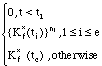where ti denotes the instant at which the ith sample of Kf*(t) has been taken, i = 1, 2, ..., e; and {Kf*(ti)}ni stands for the set composed by ni times the element Kf*(ti) such that: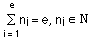.

Corollary 2

Let Kf*(t) be a finite, cumulative knowledge function until the instant t, and let te be the time of elicitation for Kf*(t).Then, the function Kf*(t) has e points at most, where Kf*(t) is non-continuous, where e corresponds to the sample of Kf*(t) at which te has been reached.

Proof It is obvious if we take into account that Kf*(tj) >= Kf*(ti), 1 <= i < j <= e.

3.3 The Knowledge Functions Calculation (KFC) Algorithm

In the previous section, we have established, in a step-by-step process, a mathematical knowledge function to characterise the existence of a finite instant at which the knowledge necessary to solve a particular KE-solvable problem (P) is reached. Based on this we are able to describe a very simple algorithm to find out the value of te and, hence, of Kf*(te).

Let En be a non-empty set of experts who participate in a KEP, and let KAT be a function standing for the available knowledge analysis techniques to be applied to the elicited expert knowledge (i.e., to be applied to its argument) required to solve P. The KFC algorithm can be written as follows:

KFC(En, KAT, operative_knowledge, te)

```begin
sample_number = 0;
repeat
sample_number = sample_number + 1;
current_knowledge = KAT (Kc(tsample_number)Kr(tsample_number));
until validation(current_knowledge, En);
te = tsample_number;
operative_knowledge = Kf*(te)
end
```
where validation is a Boolean function which returns true if the set of all available experts (Nexp) validates a knowledge base (current_KB). More precisely:

validation(current_KB, Nexp)

```begin
expert_number = 0
status = OK;
repeat
expert_number = expert_number + 1;
status = check(current_KB, expert_number)
until (expert_number = Nexp + 1) or status = false;
end;
return status
```
where the function check can be defined as follows:

check =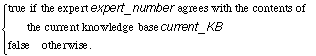### 4 Deriving Formal Parameters to Compare Knowledge Elicitation Techniques

In this section, we use the mathematical results obtained to derive relevant parameters for comparing knowledge elicitation techniques for solving KE-solvable problems.

Parameter 1: delay factor Let tei and tej be the times of elicitation corresponding to two finite, cumulative knowledge functions obtained after applying two knowledge elicitation techniques Mi and Mj respectively for solving a KE-solvable problem, written P. Mi is said to delay the KE process for solving P less (more) than Mj does if tei < (>) tej. The technique with the smallest delay factor is the preferred one.

Parameter 2: complexity of the technique We can also consider the amount of knowledge corresponding to the time of elicitation obtained after applying each of the available elicitation techniques. Thus, the number of concepts and relationships affecting them, obtained after using a particular knowledge elicitation technique, have an influence on what can be entailed from it (Lehmann and Magidor, 1992) as well as on the presence of inconsistencies (Goldszmidt and Pearl, 1991) within that knowledge.

Let Kfi*(t) and Kfj*(t) be two finite, cumulative knowledge functions obtained after applying two different knowledge elicitation techniques Mi and Mj, respectively to solve a KE-solvable problem P; and let tei and tej be the times of elicitation corresponding to each of those functions. Mi is said to introduce more (less) complexity into the KE process than Mj does if Kfi*( tei) >(<) Kfj*( tej). The above definition, however, only takes into account the final value of Kf*(t) in order to evaluate the complexity associated to a particular knowledge elicitation methodology.

We can also separately consider the complexity related to the number of concepts, and to the amount of knowledge referring to the concepts (i.e., characteristics and taxonomic relationships involving concepts earlier elicited). This is the motivation for the following two definitions:

Parameter 3: concept complexity of the technique Let [rho]ci and [rho]cj be the average density of elicited concept knowledge obtained after applying the knowledge elicitation methodologies Mi and Mj ,respectively, to the same problem. Mi is said to be more (less) concept complex than Mj is if [rho]ci >(<) [rho]cj.

Parameter 4: non-concept complexity of the technique Let [rho]nci and [rho]ncj be the density of elicited non-concept knowledge elicited obtained after applying the knowledge elicitation methodologies Mi and Mj, respectively, to the same problem. Mi is said to be more (less) non-concept complex than Mj is if [rho]nci >(<) [rho]ncj.

By making an analogy with physics, a number of additional parameters can be derived.

Parameter 5: speedup Let Kf*(t) be a finite, cumulative knowledge function until the instant t. Then, the speedup of knowledge elicitation at t, written SE(t), can be defined as follows: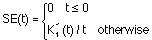Parameter 6: acceleration Let SE(t) be the speedup of the knowledge elicitation at the instant t. Then, the acceleration of the knowledge elicitation at the instant t, written AE(t), can be defined as:Parameter 7: sleeping time Another perspective of the KEP is to consider the period of time in which there is no gain of relevant knowledge for solving the KE-solvable problem under consideration. Let Kf*(t) be a finite, cumulative knowledge function until the instant t, and let te be the time of elicitation for Kf*(t). Then, the sleeping time for Kf*(t), written St, is defined as follows: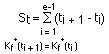Parameter 8: effective elicitation time By using the above parameter, we can determine the effective time of knowledge elicitation, that is, the period in which there has been a continuous gain of knowledge, relevant for solving a certain problem. Let Kf*(t) be a finite, cumulative knowledge function until the instant t, te the time of elicitation for Kf*(t) and St the sleeping time for Kf*(t). Then, the time of effective elicitation for Kf*(t), written EET, is defined as the difference te - St.

Parameter 9: laziness/activeness Let Kfi*(t) and Kfj*(t) be two finite, cumulative knowledge functions obtained after applying two different knowledge elicitation techniques Mi and Mj, respectively to solve a KE-solvable problem P; and let EETi and EETj be the respective times of effective elicitation corresponding to these functions. Then, Mi is said to be lazier than Mj is if EETi < EETj. In a similar way, Mi is said to be more active than Mj is if EETi < EETj.

Parameter 10: relative time of effective elicitation Elaborating on the previous parameter, we can consider the time of effective elicitation compared to the total elicitation time of each technique. Let Kf*(t) be a finite, cumulative knowledge function until the instant t, te the time of elicitation for Kf*(t) and EET the elicitation effective time for Kf*(t). Then, the relative time of effective elicitation for Kf*(t), written REET, is defined as the quotient EET/te.

Parameter 11: relative laziness/activeness If we consider the previous definition, another parameter for comparing knowledge elicitation techniques can be derived. Let REETi and REETj be the relative times of effective elicitation corresponding to two knowledge elicitation techniques Mi and Mj respectively. Mi is said to be relatively lazier than Mj if REETi < REETj.

### 5 An Example: The Landscape Study.

In this section, we present the problem for which we have performed the knowledge elicitation process. The problem is known as a natural resources planning task and the project has been carried out in co-operation with the Spanish Council for Scientific Research (CSIC). Nowadays, natural resources planning studies become more and more important, at least in the western world. To accomplish this kind of studies, AI techniques are gaining popularity in the environmental planning community. So far, several KBSs and expert systems prototypes have been developed (Starfield and Bleloch, 1983; Hunt, Middleton, Grime, and Hodgson, 1991; Martinez-Bejar, Castillo, and Martin, 1995).

Natural resources planning embodies a sub-task called physical media study which consists of performing a number of tasks, including the risks study, the fauna study, the vegetation study, the geology study, the water resources study, and the landscape study, given a particular geographic area under study (called polygon). In its turn, the polygon is usually divided into units.

Each sub-task belonging to the physical media study may be characterised as a polygonal-units assessment task, that is, as a polygonal-units classification task (Wielinga, Schreiber, and Breuker, 1992). This task is carried out by means of certain descriptive variables whose nature depends on each sub-task. Thus, the objective of the landscape study task is to make an assessment of each of the landscape units in the polygon from a landscape point of view. The assessment is typically performed by means of the following two parameters, which need to be found for each of the landscape units:

Visual Quality (VQ) of a landscape is defined as the beauty grade from the vegetation-uses, the geology-geomorphology, and the hydrology points of view simultaneously.

Visual Fragility (VF) of a landscape refers to its sensitivity to changes caused by human activities.

Basically, the goal of the landscape study task consists of obtaining a binomy B = (VQ, VF) for each landscape unit in the polygon under study. Figure 1 shows a polygon composed of 7 units assessed from the landscape point of view. The natural numbers into brackets represent the values for VQ and VF, respectively.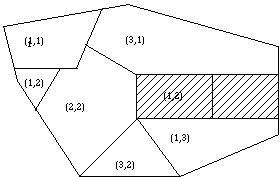FIGURE 1 - A polygonal area showing the results of performing the landscape study task

5.1 Landscape study: a KE-solvable problem?

In order to apply the results obtained in this paper, we need to ensure that the landscape study task is a KE-solvable problem. In other words, we need to ensure that the assumptions (see Section 2) hold.

Assumption 1 The problem (P) to be solved consists of determining both the visual quality and the visual fragility of a landscape. It implies to assess both parameters for a particular landscape from different point of views, including vegetation-uses, water resources, and geology-geomorphology. Each point of view is considered as a separate task. So, assumption 1 is satisfied.

Assumption 2 There have been 10 experts available to elicit the knowledge required to solve P, which is in accordance with assumption 2.

Assumption 3 In the landscape project, we have restricted the `world' of elicitation techniques to two of them: the structured interview and the unstructured interview. So, assumption 3 also holds.

Assumption 4 The elicitor has been the same person during the whole KEP. So, assumption 4 is satisfied as well.

Assumption 5 Prior to each elicitation session, the experts were given a manuscript containing the knowledge elicited until that moment in order to ensure that the elicitation session to be performed did not invalidate the knowledge obtained so far. In addition, after each elicitation session, the experts validated the knowledge elicited with respect to the previous elicitation sessions. In this way, assumption 5 is also true.

Thus, we can conclude that P is KE-solvable.

5.2 Applying the KFC algorithm

In this section, we apply the KFC algorithm to solve P. As mentioned before, there are two candidate elicitation techniques to be tested in the context of solving P. After applying the KFC algorithm to each of them, the next tables were obtained:

 t Card(Kc(t)) Card(Kr(t)) pc(t) pnc(t) K*(t) SE(t) AE(t) 15 8 14 0.363636364 0.636363 22 1.466666 0.097777 30 10 18 0.357142857 0.642857 28 0.933333 0.031111 45 10 24 0.294117647 0.705882 34 0.755555 0.016790 60 13 28 0.317073171 0.682926 41 0.683333 0.011388 75 13 31 0.295454545 0.704545 44 0.586666 0.007822 90 19 39 0.327586207 0.672413 58 0.644444 0.007160 105 21 47 0.308823529 0.691176 68 0.647619 0.006167 120 24 51 0.32 0.68 75 0.625 0.005208 135 25 59 0.297619048 0.702380 84 0.622222 0.004609 150 27 65 0.293478261 0.706521 92 0.613333 0.004088 165 31 79 0.281818182 0.718181 110 0.666666 0.004040 180 32 92 0.258064516 0.741935 124 0.688888 0.003827 195 32 101 0.240601504 0.759398 133 0.682051 0.003497 210 32 106 0.231884058 0.768115 138 0.657142 0.003129 225 32 106 0.231884058 0.768115 138 0.613333 0.002725 240 33 110 0.230769231 0.769230 143 0.595833 0.002482 255 33 112 0.227586207 0.772413 145 0.568627 0.002229 270 33 112 0.227586207 0.772413 145 0.537037 0.001989 285 38 135 0.219653179 0.780346 173 0.607017 0.002129 300 40 154 0.206185567 0.793814 194 0.646666 0.002155 315 41 165 0.199029126 0.800970 206 0.653968 0.002076 330 47 190 0.198312236 0.801687 237 0.718181 0.002176 345 49 199 0.197580645 0.802419 248 0.718840 0.002083 360 50 201 0.199203187 0.800796 251 0.697222 0.001936 375 51 209 0.196153846 0.803846 260 0.693333 0.001848 390 51 217 0.190298507 0.809701 268 0.687179 0.001761 405 51 220 0.188191882 0.811808 271 0.669135 0.001652 20 52 223 0.189090909 0.810909 275 0.654761 0.001558 435 52 225 0.187725632 0.812274 277 0.636781 0.001463 450 52 228 0.185714286 0.814285 280 0.622222 0.001382

Table 1. The value of some parameters for the structured interview method (M1).

 t Card(Kc(t)) Card(Kr(t)) pc(t) pnc(t) K*(t) SE(t) AE(t) 15 3 9 0.25 0.75 12 0.8 0.053333 30 7 15 0.318181818 0.681818 22 0.733333 0.024444 45 9 17 0.346153846 0.653846 26 0.577777 0.012839 60 10 20 0.333333333 0.666666 30 0.5 0.008333 75 10 22 0.3125 0.6875 32 0.426666 0.005688 90 12 26 0.315789474 0.684210 38 0.422222 0.004691 105 14 36 0.28 0.72 50 0.476190 0.004535 120 15 41 0.267857143 0.732142 56 0.466666 0.003888 135 15 45 0.25 0.75 60 0.444444 0.003292 150 16 49 0.246153846 0.753846 65 0.433333 0.002888 165 18 67 0.211764706 0.788235 85 0.515151 0.003122 180 18 75 0.193548387 0.806451 93 0.516666 0.002870 195 19 81 0.19 0.81 100 0.512820 0.002629 210 19 82 0.188118812 0.811881 101 0.480952 0.002290 225 19 83 0.18627451 0.813725 102 0.453333 0.002014 240 20 85 0.19047619 0.809523 105 0.4375 0.001822 255 24 100 0.193548387 0.806451 124 0.486274 0.001906 270 25 105 0.192307692 0.807692 130 0.481481 0.001783 285 26 120 0.178082192 0.821917 146 0.512280 0.001797 300 26 138 0.158536585 0.841463 164 0.546666 0.001822 315 27 142 0.159763314 0.840236 169 0.536507 0.001703 330 28 151 0.156424581 0.843575 179 0.542424 0.001643 345 30 163 0.155440415 0.844559 193 0.559420 0.001621 360 31 168 0.155778894 0.844221 199 0.552777 0.001535 375 32 171 0.157635468 0.842364 203 0.541333 0.001443 390 34 179 0.159624413 0.840375 213 0.546153 0.001400 405 35 187 0.157657658 0.842342 222 0.548148 0.001353 420 36 191 0.158590308 0.841409 227 0.540476 0.001286 435 37 202 0.154811715 0.845188 239 0.549425 0.001263 450 38 205 0.156378601 0.843621 243 0.54 0.0012 465 38 205 0.156378601 0.843621 243 0.522580 0.001123 480 38 206 0.155737705 0.844262 244 0.508333 0.001059 495 38 207 0.155102041 0.844897 245 0.494949 0.000999 510 40 209 0.16064257 0.839357 249 0.488235 0.000957 525 41 210 0.163346614 0.836653 251 0.478095 0.000910 540 41 212 0.162055336 0.837944 253 0.468518 0.000867

Table 2. The value of some parameters for the unstructured interview method (M2).

Examining the tables, we see that the problem P possesses a finite, cumulative function (Kf*(t)) whose time of elicitation (te) is 450 minutes and 540 minutes respectively for the structured interview and the unstructured interview

At this point, we can obtain all parameters defined in Section 4. Some of these are indicated in Table 3, where M1 and M2 respectively stand for the structured interview and the unstructured interview.

 te Kf*( te) pc pnc SEm AEm REET M1 450 280 0.247 0.751 0.686 0.0079 0.93 M2 540 253 0.203 0.796 0.517 0.0046 1

Table 3. Calculation of some parameters for comparing M1 and M2.

By looking at the information in the above tables, and by taking into account the contents of the previous section, we can obtain several useful results for P and the family (class) of problems to which P belongs. Some of these results are the following:

1. The KEP with M2 takes 20% longer than with M1. So, M2 is lazier than M1.

2. M1 introduces more complexity in the KEP than M2 does, because the amount of knowledge obtained after applying M1 is over 9% more than the amount after applying M2.

3. The average density of concept knowledge is higher for M1, the average density of non-concept knowledge being, hence, lower for M1.

4. The average speedup of knowledge elicitation, as well as the average acceleration of knowledge elicitation is higher for M1.

5. The sleeping time for M2 is zero, which implies that its relative time of effective elicitation (REET) is 100%. With M1, 93% of the time dedicated to the KEP is used to elicit relevant knowledge.

In other words, M1 is less lazy, more concept-complex and more dynamic (i.e., its speedup and its acceleration are higher) than M2, although M1 introduces a little more complexity than M2 does, and the REET corresponding to M1 is near to that of M2. So, it seems that the structured interview method is better than the unstructured one for this kind of problem, under the assumptions pointed out previously.

### 6 Conclusions

In real world applications, the knowledge elicitation process is a time consuming and hence influential cost-determining factor for developing knowledge-based systems. Comparing elicitation techniques formally is an interesting endeavour because it enables us to select the best elicitation technique in a particular situation. This might lead to lower development costs.

The knowledge elicitation process is influenced by various factors, including the number of experts, the kind of problem, the context in which the elicitation process takes place, and even the person who elicits the knowledge by interacting with the experts. In order to formally compare these techniques, we propose a simple semantics based on the following assumptions: (1) there are different tasks involved in the problem for which a KBS has to be developed, (2) there are various experts participating in that project (at least one for each task), (3) every current knowledge elicitation technique is available, (4) the person who elicits the knowledge is the same during the entire project, and (5) the knowledge elicitation sessions do not invalidate knowledge elicited in previous sessions.

For problems that satisfy these assumptions, and where the knowledge is limited to concepts and some well-defined relationships between them, we defined, in a step-by-step process, a set of mathematical functions which operate on cumulative knowledge. Moreover, by sampling the values corresponding to these functions during the knowledge elicitation process, a number of formal parameters are suggested which can be used for comparing different knowledge elicitation techniques.

To illustrate the usefulness of the mathematical artefacts designed in this study, we showed an example where the mentioned parameters are applied to compare the structured interview with the unstructured one. Both techniques have been applied to a real problem involving environmental planning by assessment in Spain. The results demonstrate that the structured interview method is more appropriate than the unstructured one under the assumptions pointed out above and for the assessment problem that we were concerned with.

Although, in this study, we have compared only two elicitation techniques, and the final result is not a surprise (i.e., the structured interview is more efficient than the unstructured one), we believe that the formal parameters can be used to compare any two elicitation techniques, provided that the problem at hand satisfies the identified assumptions (i.e., is KE-solvable).

### Acknowledgements

This work has been supported by an institutional research grant from the Spanish Council for Scientific Research (CSIC) and by the Netherlands Computer Science Research Foundation with financial support from the Netherlands Organisation for Scientific Research (NWO).

### References

>Bell, J., and Hardiman, R. J. (1989). The third role - the naturalistic knowledge engineer, in D. Diaper (Eds.), Knowledge engineering: Principles, techniques, and applications. Chichester: Ellis Horwood Ltd.

Berry, D. C. (1987). The problem of implicit knowledge, Expert Systems, Vol. 4:144-151.

Burton, A. M., Shadbolt, N. R., Rugg, G., and Hedgecock, A. P. (1990). The Efficacy of Knowledge Elicitation Techniques: a Comparison Across Domains and Levels of Expertise, Knowledge Acquisition, Vol. 2: 167-178.

Kang, H. B., Compton, P., and Preston, P. (1995). Multiple classification ripple down rules: evaluation and possibilities, in B. R. Gaines, and M. Musen (Eds.), Proceedings of the Ninth Banff Knowledge Acquisition for Knowledge-Based Systems Workshop, Vol. 1:17.1-17.20.

Cooke, N. J. (1994).Varieties of knowledge elicitation techniques, International Journal of Human-Computer Studies, Vol. 41:801-849.

Gammack, J. (1992). Knowledge engineering issues for decision support, in G. Wright, and F. Bolger (Eds.), Expertise and Decision Support, 203-226, Plenum Press.

Gisolfi, A., and Di Lascio, L. (1995). POTCLAS: a fuzzy expert system for the classification of archaeological pottery fragments, International Journal of Expert Systems, Vol. 8, No. 2 :145-164.

Goldszmidt, M., and Pearl, J. (1991). On the consistency of defeasible databases, Artificial Intelligence, Vol. 52:121-149.

Hart, A. (1986). Knowledge Acquisition for expert systems, Tiptree, Essex: Anchor Brendon Ltd.

Hunt, R., Middleton, D. A. J., Grime, J. P., and Hodgson, J. G. (1991). TRISTAR: an expert system for vegetation processes, Expert Systems, Vol. 8: 219-226.

Hwang, G-J. (1995). Knowledge acquisition for fuzzy expert systems, International Journal of Intelligent Systems, Vol. 10:541-560.

Lehmann, D., and Magidor, M. (1992). What does a conditional knowledge base entail?, Artificial Intelligence, Vol. 55:1-60.

Marcus, S. (1988). Automating knowledge acquisition for expert systems, Boston: Kluwer Publisher.

Martinez-Bejar, R., Castillo, V. M., and Martin, F. (1995). A knowledge-based approach for landscape study in natural resources physical planning (in Spanish), in R. Rizo, and J. M. Garcia (Eds.), Technological Transference of Artificial Intelligence to Industry, Medicine and Social Applications, 209-216, Universidad de Alicante.

MOPT. (1992). A Guidebook for making physical environment studies (in Spanish), MOPU, Madrid: Secretaría de Estado para las Políticas del Agua y el Medio Ambiente.

Nisbett, R. E., and Wilson, T. D. (1977). Telling more than we can know: verbal reports on mental processes, Psychological Review, Vol. 84:231-259.

O'Hara, K., Motta, E., and Shadbolt, N. (1994). Grounding GDMs: A Structured Case Study, International Journal of Human-Computer Studies, Vol. 40: 315-347.

Puerta, A. R., Egar, J., Tu, S., and Musen, M. (1992). A Multiple-Method Shell for the Automatic Generation of Knowledge Acquisition Tools, Knowledge Acquisition, Vol. 4:171-196.

Runkel, J. T., and Birmingham, W. P. (1993). Knowledge acquisition in the small: building knowledge-acquisition tools from pieces, Knowledge Acquisition, Vol. 5:221-243.

Starfield, A. M., and Bleloch, A. L. (1983). Expert Systems: An approach to problems in ecological management that are difficult to quantify, Journal of Environmental Management, Vol. 16: 261-268.

Welbank, M. (1983). A review of knowledge acquisition techniques for expert systems, British Telecommunications Research Laboratories Technical Report, Ipswich, England: Martlesham Heath.

Wielinga, B. J., Schreiber, A. T., and Breuker, J. A. (1992). KADS: a modelling approach to knowledge engineering, Knowledge Acquisition, Vol. 4:5-53.

 The term visual fragility of a landscape refers to its sensitivity to changes caused by human activities (MOPT, 1992).

 Decision rules are the rules the expert uses to solve the problem. Note that, although we use (production) rules in the formalisation, the rule format as such is not essential. What is essential, is some kind of carrier of concepts and relations. In this paper, we use rules because the experts involved in the case-study expressed themselves through rules.

 The term "concepts" comprises the relevant objects needed to represent and solve the problem.

 For an overview of the different ways in which experts can participate in the KEP, see Cooke (1994).

 We assume that experts participating in a KEP effectively can detect non-relevant knowledge whenever needed.

 In this article, landscape study and landscape study task are used as synonyms.